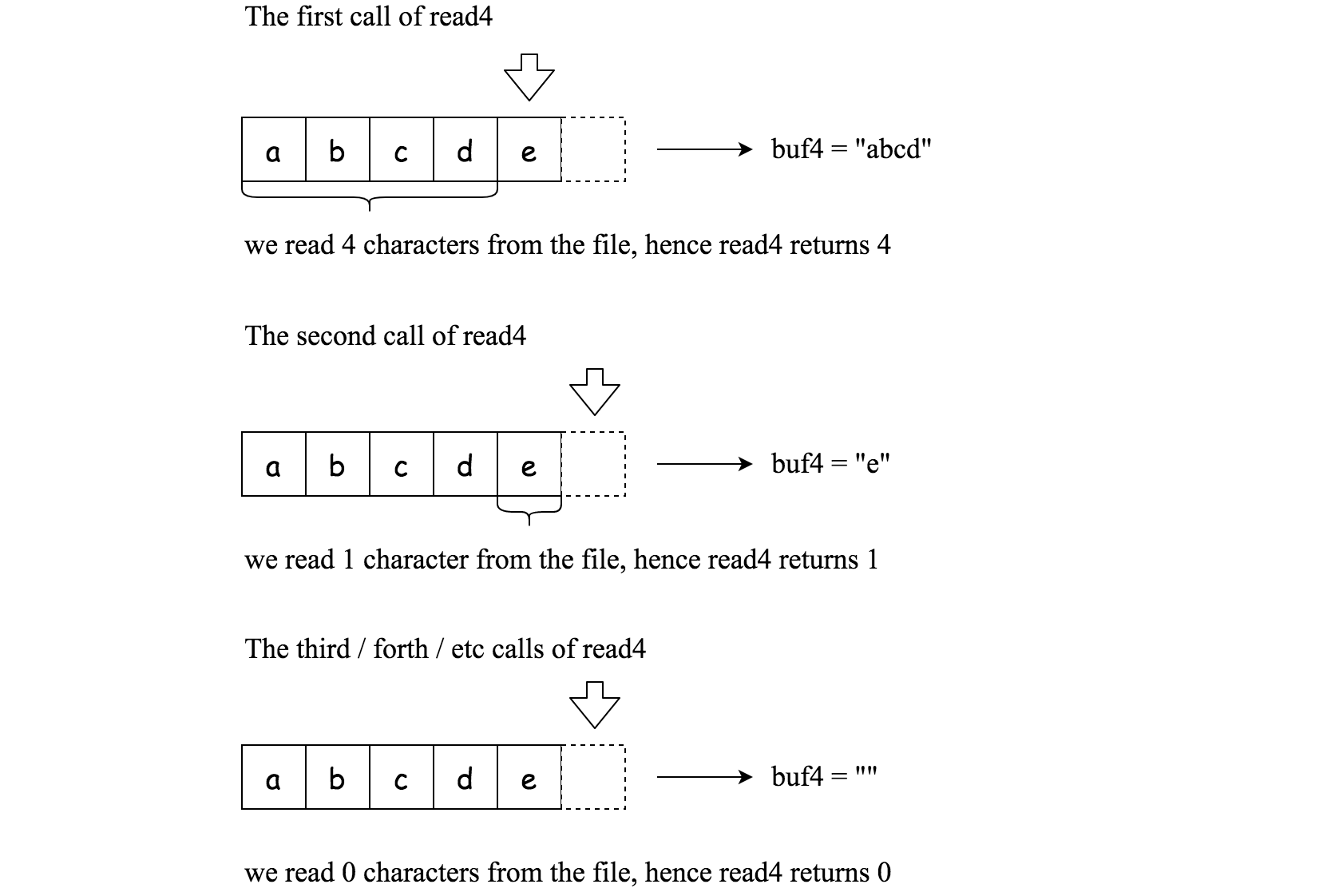Given a file and assume that you can only read the file using a given method `read4`, implement a method to read n characters.

The API `read4` reads 4 consecutive characters from the file, then writes those characters into the buffer array `buf4`.

The return value is the number of actual characters read.

Note that `read4()` has its own file pointer, much like `FILE *fp` in C.

```    Parameter:  char[] buf4
Returns:    int

Note: buf4[] is destination not source, the results from read4 will be copied to buf4[]
```

Below is a high level example of how `read4` works:``````File file("abcde"); // File is "abcde", initially file pointer (fp) points to 'a'
char[] buf4 = new char; // Create buffer with enough space to store characters
read4(buf4); // read4 returns 4. Now buf4 = "abcd", fp points to 'e'
read4(buf4); // read4 returns 1. Now buf4 = "e", fp points to end of file
read4(buf4); // read4 returns 0. Now buf4 = "", fp points to end of file```
```

By using the `read4` method, implement the method `read` that reads n characters from the file and store it in the buffer array `buf`. Consider that you cannot manipulate the file directly.

The return value is the number of actual characters read.

```    Parameters:	char[] buf, int n
Returns:	int

Note: buf[] is destination not source, you will need to write the results to buf[]
```

Example 1:

```Input: file = "abc", n = 4
Output: 3
Explanation: After calling your read method, buf should contain "abc". We read a total of 3 characters from the file, so return 3. Note that "abc" is the file's content, not buf. buf is the destination buffer that you will have to write the results to.
```

Example 2:

```Input: file = "abcde", n = 5
Output: 5
Explanation: After calling your read method, buf should contain "abcde". We read a total of 5 characters from the file, so return 5.
```

Example 3:

```Input: file = "abcdABCD1234", n = 12
Output: 12
Explanation: After calling your read method, buf should contain "abcdABCD1234". We read a total of 12 characters from the file, so return 12.
```

Example 4:

```Input: file = "leetcode", n = 5
Output: 5
Explanation: After calling your read method, buf should contain "leetc". We read a total of 5 characters from the file, so return 5.
```

Note:

• Consider that you cannot manipulate the file directly, the file is only accesible for `read4` but not for `read`.
• The `read` function will only be called once for each test case.
• You may assume the destination buffer array, `buf`, is guaranteed to have enough space for storing n characters.

``````use std::cell::RefCell;
use std::rc::Rc;

struct Solution {
file: Vec<char>,
index: Rc<RefCell<usize>>,
}

impl Solution {
fn new(file: String) -> Self {
let file = file.chars().collect();
let index = Rc::new(RefCell::new(0));
Solution { file, index }
}

fn read4(&self, buf4: &mut [char]) -> i32 {
for i in 0..4 {
if *self.index.borrow() == self.file.len() {
return i as i32;
}
buf4[i] = self.file[*self.index.borrow()];
*self.index.borrow_mut() += 1;
}
4
}
}

impl Solution {
fn read(&self, buf: &mut [char], n: i32) -> i32 {
let n = n as usize;
let mut buf4: Vec<char> = vec![' '; 4];
let mut i = 0;
let mut j = 0;
let mut m = 0;
while i < n {
if m == 0 {
j = 0;
if m == 0 {
break;
}
} else {
buf[i] = buf4[j];
i += 1;
j += 1;
m -= 1;
}
}
i as i32
}
}

#[test]
fn test() {
let obj = Solution::new("abc".to_string());
let n = 4;
let mut buf = vec![' '; 100];
let res = 3;
let obj = Solution::new("abcde".to_string());
let n = 5;
let mut buf = vec![' '; 100];
let res = 5;